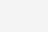# npv计算公式（净现值npv公式解释）

CFA一级重难点净现值（NPV）和内部收益率（IRR）是进行项目投资时非常常见也非常重要的财务指标，那么项目是否值得投资的重要参考数据。在这两个指标之外，还衍生出了持有期回报（HPR）、货币加权回报（Money-weighted Return）和时间加权回报（Time-weighted Return）等概念。

CFA一级重难点：NPV和各种回报率的计算CFA一级npv公式

NPV = -100,000 + 0 + 0 + 0 + 30,000/(1+6%)^4 + 50,000/(1+6%)^5 + 70,000/(1+6%)^6 + 40,000/(1+6%)^7

CF0 = -100,000; CF1 = 0; CF2 = 0; CF3 = 0; CF4 = +30,000; CF5 = +50,000;

CF6 = +70,000; CF7 = +40,000

NPV, I = 6, CPT, NPV = 37,075

NPV明显大于零，说明该项目值得投资。

NPV判断法则：接受NPV大于零的项目;拒绝NPV小于零的项目;如果两个项目的NPV都为正，则接受NPV更大的项目。

CF0 = -100,000; CF1 = 0; CF2 = 0; CF3 = 0; CF4 = +30,000; CF5 = +50,000;

CF6 = +70,000; CF7 = +40,000

IRR判断法则：接受IRR大于要求的回报率的项目，拒绝IRR小于要求的回报率的项目。HPR = (Ending Value + cash flow received – beginning value)/beginning value

= (55,000 + 500 – 50,000)/50,000

= 11%

0 = PV outflows + PV inflows

0 = – 50,000 + 500/(1+IRR) + 55,000/(1+IRR)^2，或者

50,000 = 500/(1+IRR) + 55,000/(1+IRR)^2

Time-weighted Return的计算就要用到前面讲过的持有期回报HPR的概念，先分别计算每个阶段的额回报率，在计算整个期间的回报率水平即可。

2016年的HPR = 500/50,000 = 1%

2017年的HPR = (55,000 – 50,000)/50,000 = 10%

(1+Time-weighted Return)^2 = (1+1%)*(1+10%)，求解，得到Time-weighted Return = 5.40%，即这里的Time-weighted Return就是5.40%。1、本文内容及相关资源来源于网络，版权归版权方所有！本站原创内容版权归本站所有，请勿转载！发布者：笑笑考吧，转转请注明出处：https://xiaofangkb.com/14442.html

2、本文内容仅代表作者本人观点，不代表本网站立场，作者文责自负，本站资源仅供学习研究，请勿非法使用，否则后果自负！请下载后24小时内删除！

3、本文内容、文字、图片等，仅供参考使用，本站不对其安全性，正确性等作出保证。但本站会尽量审核会员发表的内容

4、如您认为本文内容侵犯了您的权益，请与我们联系！我们将在5个工作日内做出处理！本站保留全部修改、解释、更新本声明的权利

(1)

05.0K0

02700

05880

05000

02.6K0

02.3K0